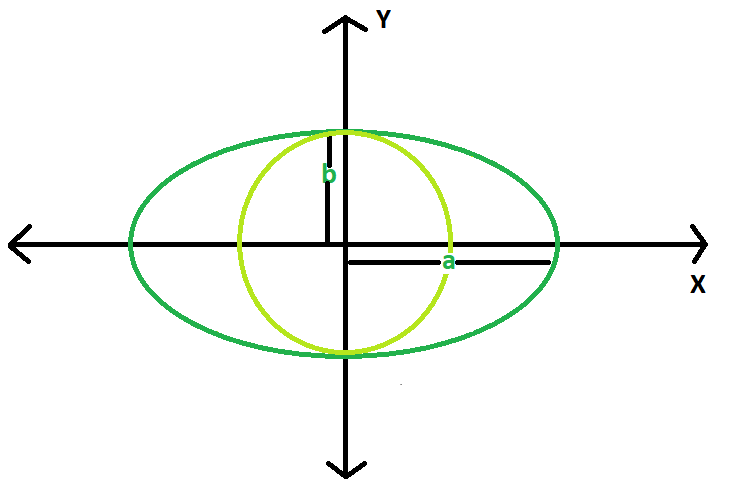Find the area of largest circle inscribed in ellipse

• Last Updated : 18 Mar, 2021

Given an ellipse, with major and minor axis length 2a & 2b respectively. The task is to find the area of the largest circle that can be inscribed in it.
Examples:

Input : a = 5, b = 3
Output : 28.2743

Input : a = 10, b = 8
Output : 201.062Approach : The maximal radius of the circle inscribed in the ellipse is the minor axis of the ellipse.
So, area of the largest circle = π * b * b.
Below is the implementation of the above approach:

C++

 // CPP program to find// the area of the circle#include using namespace std;#define pi 3.1415926 double areaCircle(double b){    double area = pi * b * b;    return area;} // Driver Codeint main(){    double a = 10, b = 8;    cout << areaCircle(b);    return 0;}

Java

 // Java Program to find the area// of circle class GFG{    static double areaCircle(double b)    {                  // Area of the Reuleaux triangle        double area = (double)3.1415926 * b * b;        return area;    }         // Driver code    public static void main(String args[])    {        float a = 10,b = 8;        System.out.println(areaCircle(b)) ;    }} // This code is contributed by mohit kumar 29

Python3

 # Python3 program implementation of above approach import math # Function to return required answerdef areaCircle(b):    area = math.pi * b * b    return area  # Driver Codea = 10b = 8print(areaCircle(b)) # This code is contributed by# Sanjit_Prasad

C#

 // C# Program to find the area// of circleusing System; class GFG{    static double areaCircle(double b)    {        // Area of the Reuleaux triangle        double area = (double)3.1415926 * b * b;        return area;    }         // Driver code    public static void Main()    {        float b = 8;        Console.WriteLine(areaCircle(b)) ;    }} // This code is contributed by aishwarya.27



Javascript


Output:
201.062

My Personal Notes arrow_drop_up